### Home > AC > Chapter 14 > Lesson 14.2.1.8 > Problem2-113

2-113.
1. The right triangle shown below has a height of 8 cm and an area of 60 square cm. Complete parts (a) and (b) below, referring to the Math Notes box in Lesson 1.2.1 for further guidance, if necessary. Homework Help ✎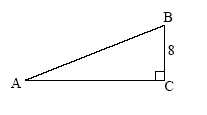1. Find the measure of ∠A to the nearest tenth.

2. Find the perimeter of the triangle.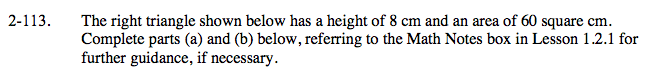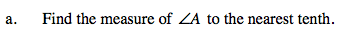Start by finding the length of the base of the triangle.

Use the tangent ratio to find the measure of angle A.

m∠A ≈ 28.07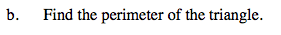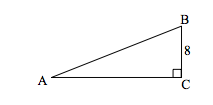Use the Pythagorean Theorem to find the length of the hypotenuse.

Perimeter = 40 cm